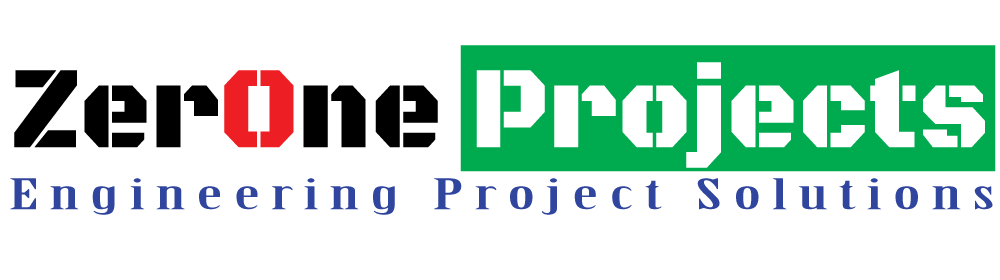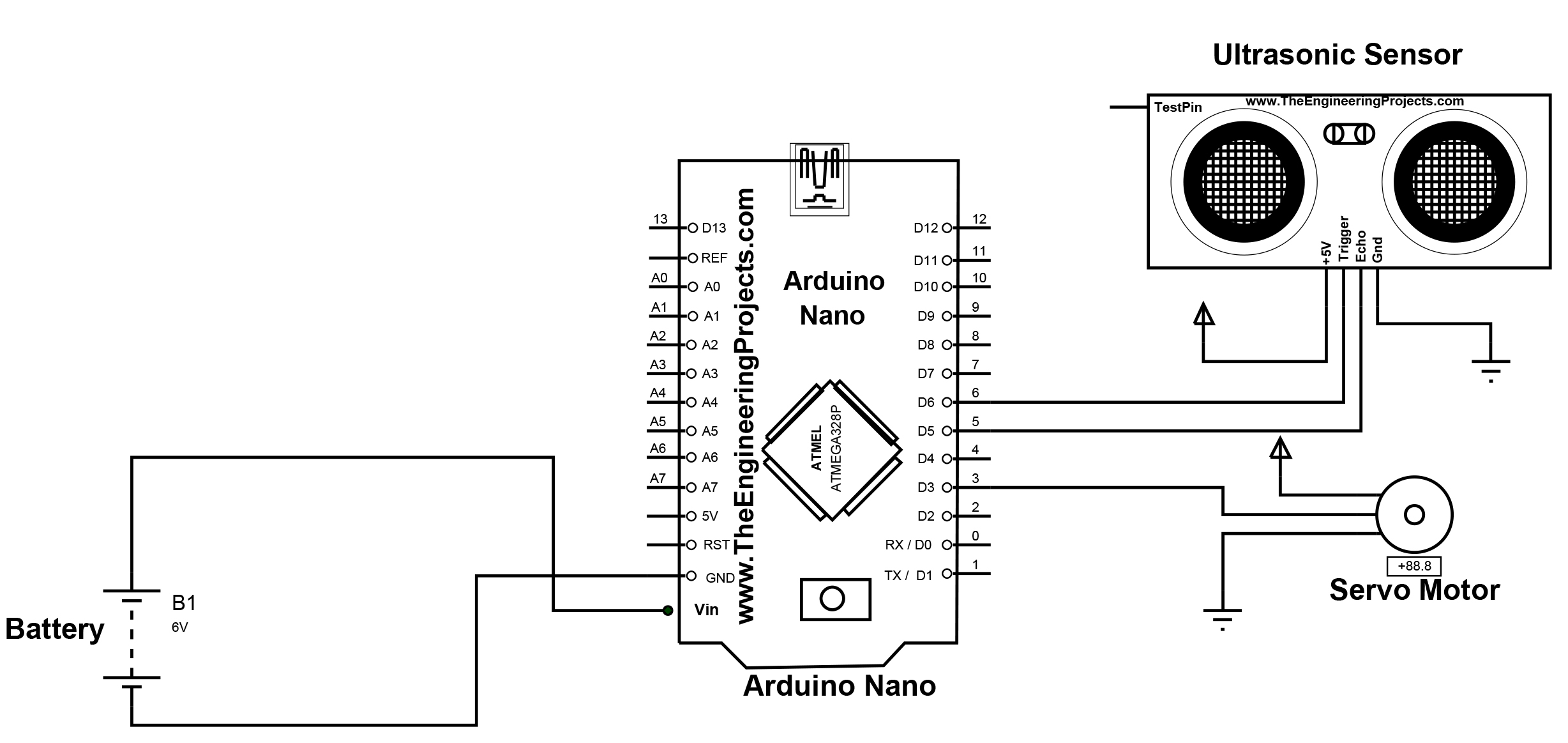Phone : +8801676998099 Email : projects.zeronebd@gmail.com
###### support 24/7## How to Make a Radar Using Arduino & Ultrasonic Sensor

How to Make a Radar Using Arduino & Ultrasonic Sensor

Project Fee:Negotiable

Project Discount:0

Project Duration:One Month

How to Make a Radar Using Arduino & Ultrasonic Sensor.

Introduction:

In this project, I will show you how to design a simple Radar Application using Arduino and Processing. This Arduino Radar Project is implemented with the help of Processing Application. Radar is a long-range object detection system that uses radio waves to establish certain parameters of an object like its range, speed and position. Radar technology is used in aircrafts, missiles, marine, weather predictions and automobiles.

Even though the title says Arduino Radar Project, technically the project is based on Sonar technology as I will be using an Ultrasonic Sensor to determine the presence of any object in a particular range. The Arduino Radar Project is more of a visual project than it is a circuit implementation. Of course, I will be using different hardware like Arduino Nano, HC-SR04 Ultrasonic Sensor and a Servo Motor but the main aspect is the visual representation in the Processing Application.

Circuit Diagram:

If you look at the circuit diagram, the design of the circuit for this project is very simple. The control pin of the servo is connected to Pin 12 of the Arduino while the TRIG and ECHO Pins of the Ultrasonic Sensor are connected to Pins 10 & 11 of Arduino respectively. A separate 5V power supply (with common GND) is given to the Servo Motor and the Ultrasonic Sensor.Figure: Circuit Diagram of Radar Using Arduino & Ultrasonic Sensor.

Required Component:

·       Arduino Nano

·       HC-SR04 Ultrasonic Sensor

·       TowerPro SG90 Servo Motor

·       Mounting Bracket for Ultrasonic Sensor (optional)

·       Connecting Wires

·       Jumper Cables

·       5V Power Supply

·       USB Cable (for Arduino)

Software

·       Arduino IDE

·       Processing Application

Code:

//*****************************ZerOne BD Ltd*************************

//*********************ZerOne Projecs ******************

//*********************ZerOne Tech******************

//            Code Developed By...

//            Engr.Md.Nazmuzzaman Jomadder

//            ZerOne BD Ltd.

//            Contact: 01676998099

//            E-mail:sumonj.eee@gmail.com

//            Office:

//            ZerOne BD Ltd.

//            Plot#03(1st Floor),Road#04,Sec#6/ka.

//            Mirpur, Dhaka-1216

//            Contact:01676998099

//            E-mail:

//            projects.zeronebd@gmail.com

//            zeronebd16@gmail.com

//            bd.zeronetech@gmail.com

//            Web:

//            www.zeronebd.com

//            www.zeronetechbd.com

//            projects.zeronebd@gmail.com

//.................................................................

//***Arduino Code***

#include <Servo.h>.

const int trigPin = 10;

const int echoPin = 11;

long duration;

int distance;

Servo myServo;

void setup() {

pinMode(trigPin, OUTPUT);

pinMode(echoPin, INPUT);

Serial.begin(9600);

myServo.attach(12);

}

void loop() {

for(int i=15;i<=165;i++){

myServo.write(i);

delay(30);

distance = calculateDistance();

Serial.print(i);

Serial.print(",");

Serial.print(distance);

Serial.print(".");

}

for(int i=165;i>15;i--){

myServo.write(i);

delay(30);

distance = calculateDistance();

Serial.print(i);

Serial.print(",");

Serial.print(distance);

Serial.print(".");

}

}

int calculateDistance(){

digitalWrite(trigPin, LOW);

delayMicroseconds(2);

digitalWrite(trigPin, HIGH);

delayMicroseconds(10);

digitalWrite(trigPin, LOW);

duration = pulseIn(echoPin, HIGH); time in microseconds

distance= duration*0.034/2;

return distance;

}

//***Processing Code***

import processing.serial.*;

import java.awt.event.KeyEvent; port

import java.io.IOException;

Serial myPort;

String angle="";

String distance="";

String data="";

String noObject;

float pixsDistance;

int iAngle, iDistance;

int index1=0;

int index2=0;

PFont orcFont;

void setup() {

size (1200, 700); // ***CHANGE THIS TO YOUR SCREEN RESOLUTION***

smooth();

myPort = new Serial(this,"COM5", 9600); // starts the serial communication

myPort.bufferUntil('.'); // reads the data from the serial port up to the character '.'. So actually it reads this: angle,distance.

}

void draw() {

fill(98,245,31);

// simulating motion blur and slow fade of the moving line

noStroke();

fill(0,4);

rect(0, 0, width, height-height*0.065);

fill(98,245,31); // green color

// calls the functions for drawing the radar

drawRadar();

drawLine();

drawObject();

drawText();

}

void serialEvent (Serial myPort) { // starts reading data from the Serial Port

// reads the data from the Serial Port up to the character '.' and puts it into the String variable "data".

data = myPort.readStringUntil('.');

data = data.substring(0,data.length()-1);

index1 = data.indexOf(","); // find the character ',' and puts it into the variable "index1"

angle= data.substring(0, index1); // read the data from position "0" to position of the variable index1 or thats the value of the angle the Arduino Board sent into the Serial Port

distance= data.substring(index1+1, data.length()); // read the data from position "index1" to the end of the data pr thats the value of the distance

// converts the String variables into Integer

iAngle = int(angle);

iDistance = int(distance);

}

void drawRadar() {

pushMatrix();

translate(width/2,height-height*0.074); // moves the starting coordinats to new location

noFill();

strokeWeight(2);

stroke(98,245,31);

// draws the arc lines

arc(0,0,(width-width*0.0625),(width-width*0.0625),PI,TWO_PI);

arc(0,0,(width-width*0.27),(width-width*0.27),PI,TWO_PI);

arc(0,0,(width-width*0.479),(width-width*0.479),PI,TWO_PI);

arc(0,0,(width-width*0.687),(width-width*0.687),PI,TWO_PI);

// draws the angle lines

line(-width/2,0,width/2,0);

line(0,0,(-width/2)*cos(radians(30)),(-width/2)*sin(radians(30)));

line(0,0,(-width/2)*cos(radians(60)),(-width/2)*sin(radians(60)));

line(0,0,(-width/2)*cos(radians(90)),(-width/2)*sin(radians(90)));

line(0,0,(-width/2)*cos(radians(120)),(-width/2)*sin(radians(120)));

line(0,0,(-width/2)*cos(radians(150)),(-width/2)*sin(radians(150)));

line((-width/2)*cos(radians(30)),0,width/2,0);

popMatrix();

}

void drawObject() {

pushMatrix();

translate(width/2,height-height*0.074); // moves the starting coordinats to new location

strokeWeight(9);

stroke(255,10,10); // red color

pixsDistance = iDistance*((height-height*0.1666)*0.025); // covers the distance from the sensor from cm to pixels

// limiting the range to 40 cms

if(iDistance<40){

// draws the object according to the angle and the distance

line(pixsDistance*cos(radians(iAngle)),-pixsDistance*sin(radians(iAngle)),(width-width*0.505)*cos(radians(iAngle)),-(width-width*0.505)*sin(radians(iAngle)));

}

popMatrix();

}

void drawLine() {

pushMatrix();

strokeWeight(9);

stroke(30,250,60);

translate(width/2,height-height*0.074); // moves the starting coordinats to new location

line(0,0,(height-height*0.12)*cos(radians(iAngle)),-(height-height*0.12)*sin(radians(iAngle))); // draws the line according to the angle

popMatrix();

}

void drawText() { // draws the texts on the screen

pushMatrix();

if(iDistance>40) {

noObject = "Out of Range";

}

else {

noObject = "In Range";

}

fill(0,0,0);

noStroke();

rect(0, height-height*0.0648, width, height);

fill(98,245,31);

textSize(25);

text("10cm",width-width*0.3854,height-height*0.0833);

text("20cm",width-width*0.281,height-height*0.0833);

text("30cm",width-width*0.177,height-height*0.0833);

text("40cm",width-width*0.0729,height-height*0.0833);

textSize(40);

text("Indian Lifehacker ", width-width*0.875, height-height*0.0277);

text("Angle: " + iAngle +" °", width-width*0.48, height-height*0.0277);

text("Distance: ", width-width*0.26, height-height*0.0277);

if(iDistance<40) {

text("        " + iDistance +" cm", width-width*0.225, height-height*0.0277);

}

textSize(25);

fill(98,245,60);

translate((width-width*0.4994)+width/2*cos(radians(30)),(height-height*0.0907)-width/2*sin(radians(30)));

rotate(-radians(-60));

text("30°",0,0);

resetMatrix();

translate((width-width*0.503)+width/2*cos(radians(60)),(height-height*0.0888)-width/2*sin(radians(60)));

rotate(-radians(-30));

text("60°",0,0);

resetMatrix();

translate((width-width*0.507)+width/2*cos(radians(90)),(height-height*0.0833)-width/2*sin(radians(90)));

rotate(radians(0));

text("90°",0,0);

resetMatrix();

translate(width-width*0.513+width/2*cos(radians(120)),(height-height*0.07129)-width/2*sin(radians(120)));

rotate(radians(-30));

text("120°",0,0);

resetMatrix();

translate((width-width*0.5104)+width/2*cos(radians(150)),(height-height*0.0574)-width/2*sin(radians(150)));

rotate(radians(-60));

text("150°",0,0);

popMatrix();

}

Radar Output :